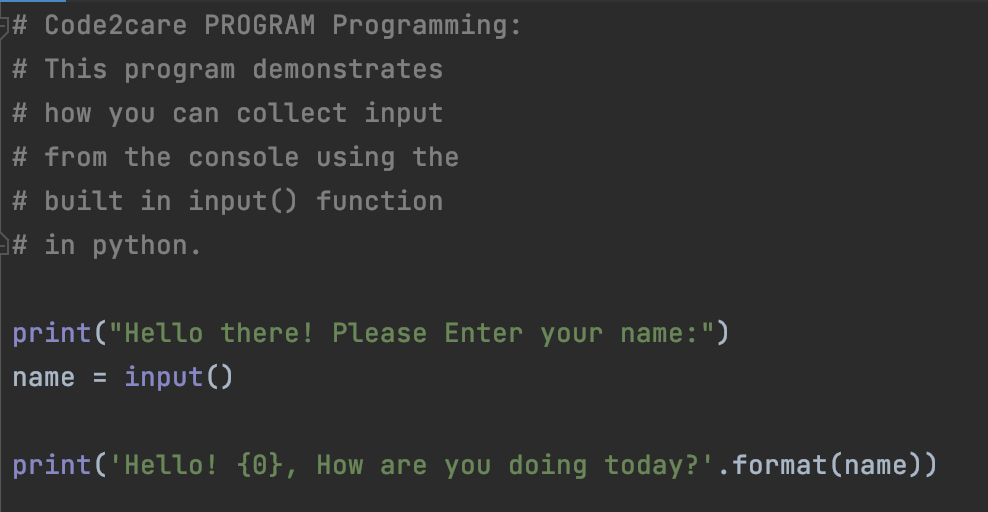# How to take user input from the console in a Python program

If you want to take inputs from the console in your Python program then you can make use of the built-in function called input()

Let's see an example where the user is prompted to enter his name and a greeting message is displayed back.

``````# Code2care PROGRAM Programming:
# This program demonstrates
# how you can collect input
# from the console using the
# built in input() function
# in python.

name = input()

print('Hello! {0}, How are you doing today?'.format(name))``````
Program Output:

Hello! Chris, How are you doing today?

Process finished with exit code 0

As you can see after the first print message the console waits for the user to input something in the console, once the user press enters the program move ahead and the inputted string is stored in the variable.Python Example to take input from console

### What if the program needs two inputs from the user?

This is easy, all you will do is make use of the input() function twice in your code wherever it is required, let's see an example where you want to add two numbers inputted by the user,

``````# Code2care PROGRAM Programming:
# Add two numbers using two numbers inputted by
# user in the console

print('This Program Adds Two Numbers inputted by the user in console:')

print('Enter 1st Number:')
number1 = int(input())

print('Enter 2nd Number:')
number2 = int(input())

sumOfNumbers = number1 + number2

print('Sum: {0} + {1} = {2}'.format(number1, number2, sumOfNumbers))``````

Note that we have typecasted the input string as an int in order to treat it as an integer.

Have Questions? Post them here!